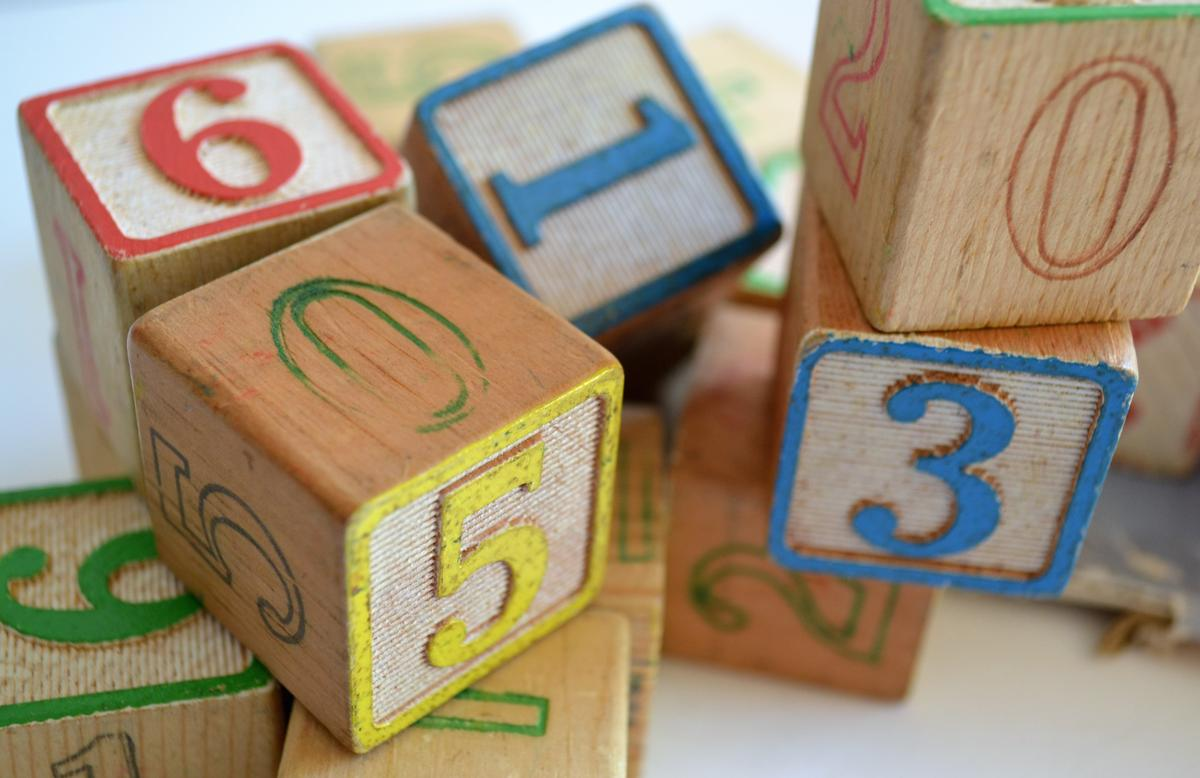Numeracy

WHAT DO WE LEARN IN MATHEMATICS?

Year Three

Dear Parents and Carers,

Over the coming weeks I will continue to outline what the teaching and learning Mathematics curriculum encompasses for each year level. In this article I will focus on Year Three. In the classroom children may be working at level, below level or above level. Teachers differentiate the teaching and tasks to cater for the learning each child needs to access.

In level 3, students increasingly use mathematical terms and symbols to describe computations. There are three major areas Number and Algebra, Measurement and Geometry, Statistics and Probability.

Number and Algebra

Number and place value

• Investigate the conditions required for a number to be odd or even and identify odd and even numbers
• Recognise, model, represent and order numbers to at least 10 000
• Apply place value to partition, rearrange and regroup numbers to at least 10 000 to assist calculations and solve problems
• Recognise and explain the connection between addition and subtraction
• Recall addition facts for single-digit numbers and related subtraction facts to develop increasingly efficient mental strategies for computation
• Recall multiplication facts of two, three, five and ten and related division facts
• Represent and solve problems involving multiplication using efficient mental and written strategies and appropriate digital technologies.

Money and financial mathematics

• Represent money values in multiple ways and count the change required for simple

Fractions and Decimals

• Model and represent unit fractions including 1/2, 1/4, 1/3, 1/5 and their multiples to a complete whole.

Patterns and algebra

• Describe, continue, and create number patterns resulting from performing addition or subtraction
• Use a function machine and the inverse machine as a model to apply mathematical rules to numbers or shapes.

Measurement and Geometry

Using units of measurement

• Measure, order and compare objects using familiar metric units of length, area, mass and capacity
• Tell time to the minute and investigate the relationship between units of time.

Shape

• Make models of three-dimensional objects and describe key features.

Location and transformation

• Create and interpret simple grid maps to show position and pathways
• Identify symmetry in the environment
• Identify and describe slides and turns found in the natural and built environment.

Geometric reasoning

• Identify angles as measures of turn and compare angle sizes in everyday situations

Statistics and Probability

Chance

• Conduct chance experiments, identify and describe possible outcomes and recognise variation in results.

Data representation and interpretation

• Identify questions or issues for categorical variables. Identify data sources and plan methods of data collection and recording
• Collect data, organise into categories and create displays using lists, tables, picture graphs and simple column graphs, with and without the use of digital technologies
• Interpret and compare data displays.

Kind regards,

Jennifer O’Connor

Assistant Principal